# Desired Profit In Units

Let's say that the owner of Oil Change Co. needs to earn a profit of \$1,200 per week rather than merely breaking even. You can consider the owner's required profit of \$1,200 per week as another fixed expense. In other words, the fixed expenses will now be \$3,600 per week (the \$2,400 listed earlier plus the required \$1,200 for the owner). The new volume required to earn \$1,200 per week is shown by the following break-even formula: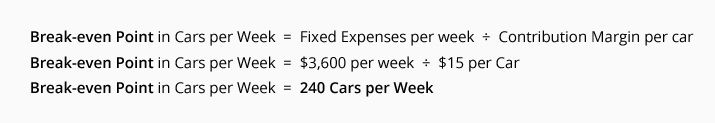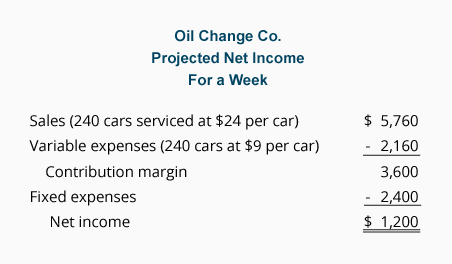The above schedule confirms that servicing 240 cars during a week will result in the required \$1,200 profit for the week.

## Break-even Point In Sales Dollars

One can determine the break-even point in sales dollars (instead of units) by dividing the company's total fixed expenses by the contribution margin ratio.

The contribution margin ratio is the contribution margin divided by sales (revenues). The ratio can be calculated using company totals or per unit amounts. We will compute the contribution margin ratio for the Oil Change Co. by using its per unit amounts: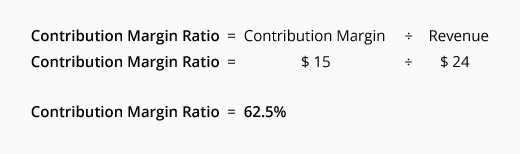The break-even point in sales dollars for Oil Change Co. is: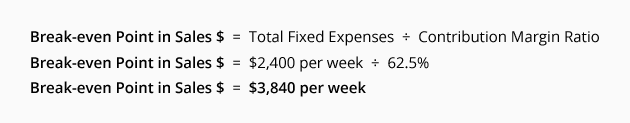The break-even point of \$3,840 of sales per week can be verified by referring back to the break-even point in units. Recall there were 160 units necessary to break even. At \$24 per unit the necessary sales in dollars would be \$3,840.

## Desired Profit In Sales Dollars

Let's assume a company needs to cover \$2,400 of fixed expenses each week plus earn \$1,200 of profit each week. In essence the company needs to cover the equivalent of \$3,600 of fixed expenses each week.

Presently the company has annual sales of \$100,000 and its variable expenses amount to \$37,500 per year. These two facts result in a contribution margin ratio of 62.5%: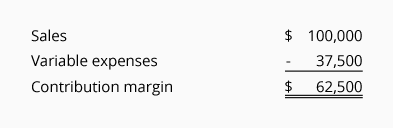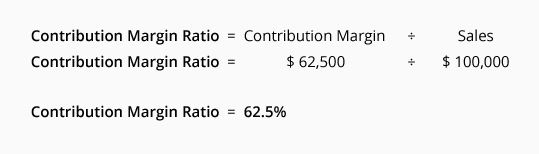The amount of sales necessary to give the owner a profit of \$1,200 per week is determined by this break-even point formula: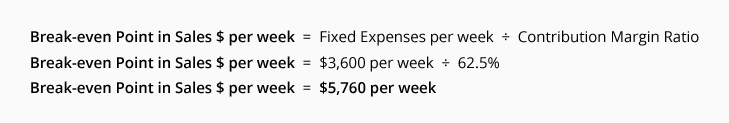To verify that this answer is reasonable, we prepared the following schedule: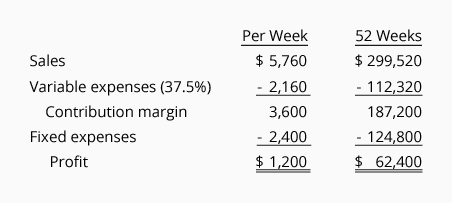As you can see, for the owner to have a profit of \$1,200 per week or \$62,400 per year, the company's annual sales must triple. Presently the annual sales are \$100,000 but the sales need to be \$299,520 per year in order for the annual profit to be \$62,400.

#### Take Our Practice Quiz

We recommend that you now take our free Practice Quiz for this topic so that you can...

• See what you know
• See what you don't know